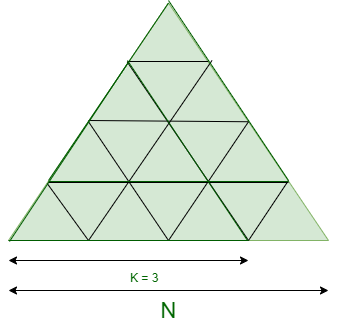# Maximum count of Equilateral Triangles that can be formed within given Equilateral Triangle

• Difficulty Level : Expert
• Last Updated : 23 Mar, 2021

Given two integers N and K where N denotes the unit size of a bigger Equilateral Triangle, the task is to find the number of an equilateral triangle of size K that are present in the bigger triangle of side N.

Examples:

Input: N = 4, K = 3Output: 3
Explanation:
There are 3 equilateral triangles of 3 unit size which are present in the Bigger equilateral triangle of size 4 units.

Input: N = 4, K = 2
Output: 7
Explanation:
There are 7 equilateral triangles of 2 unit size which are present in the Bigger equilateral triangle of size 4 units.

Naive Approach: The idea is to iterate over all possible sizes of the bigger equilateral triangle for checking the number of triangles with the required size K and print the total count of triangles.

Time Complexity: O(N)
Auxiliary Space: O(1)

Efficient Approach: To optimize the above approach, observe the following points:

• The number of triangles with a peak in the upward direction of size K present in size N equals to ((N – K +1 ) * (N – K + 2))/2.
• The number of inverted triangles with a peak in the downward direction of size K present in size N equals to ((N – 2K + 1) * (N – 2K + 2))/2.

Below is the implementation of the above approach:

## C++

 `// C++ program for the above approach` `#include ` `using` `namespace` `std;`   `// Function to find the number of` `// equilateral triangle formed` `// within another triangle` `int` `No_of_Triangle(``int` `N, ``int` `K)` `{` `    ``// Check for the valid condition` `    ``if` `(N < K)` `        ``return` `-1;`   `    ``else` `{`   `        ``int` `Tri_up = 0;`   `        ``// Number of triangles having` `        ``// upward peak` `        ``Tri_up = ((N - K + 1)` `                  ``* (N - K + 2))` `                 ``/ 2;`   `        ``int` `Tri_down = 0;`   `        ``// Number of inverted triangles` `        ``Tri_down = ((N - 2 * K + 1)` `                    ``* (N - 2 * K + 2))` `                   ``/ 2;`   `        ``// Total no. of K sized triangle` `        ``return` `Tri_up + Tri_down;` `    ``}` `}`   `// Driver Code` `int` `main()` `{` `    ``// Given N and K` `    ``int` `N = 4, K = 2;`   `    ``// Function Call` `    ``cout << No_of_Triangle(N, K);` `    ``return` `0;` `}`

## Java

 `// Java program for the above approach` `import` `java.util.*;` `class` `GFG{`   `// Function to find the number of` `// equilateral triangle formed` `// within another triangle` `static` `int` `No_of_Triangle(``int` `N, ``int` `K)` `{` `    ``// Check for the valid condition` `    ``if` `(N < K)` `        ``return` `-``1``;`   `    ``else` `    ``{` `        ``int` `Tri_up = ``0``;`   `        ``// Number of triangles having` `        ``// upward peak` `        ``Tri_up = ((N - K + ``1``) * (N - K + ``2``)) / ``2``;`   `        ``int` `Tri_down = ``0``;`   `        ``// Number of inverted triangles` `        ``Tri_down = ((N - ``2` `* K + ``1``) * ` `                    ``(N - ``2` `* K + ``2``)) / ``2``;`   `        ``// Total no. of K sized triangle` `        ``return` `Tri_up + Tri_down;` `    ``}` `}`   `// Driver Code` `public` `static` `void` `main(String[] args)` `{` `    ``// Given N and K` `    ``int` `N = ``4``, K = ``2``;`   `    ``// Function Call` `    ``System.out.print(No_of_Triangle(N, K));` `}` `}`   `// This code is contributed by PrinciRaj1992`

## Python3

 `# Python3 program for the above approach`   `# Function to find the number of` `# equilateral triangle formed` `# within another triangle` `def` `No_of_Triangle(N, K):` `  `  `    ``# Check for the valid condition` `    ``if` `(N < K):` `        ``return` `-``1``;`   `    ``else``:` `        ``Tri_up ``=` `0``;`   `        ``# Number of triangles having` `        ``# upward peak` `        ``Tri_up ``=` `((N ``-` `K ``+` `1``) ``*` `                  ``(N ``-` `K ``+` `2``)) ``/``/` `2``;`   `        ``Tri_down ``=` `0``;`   `        ``# Number of inverted triangles` `        ``Tri_down ``=` `((N ``-` `2` `*` `K ``+` `1``) ``*` `                    ``(N ``-` `2` `*` `K ``+` `2``)) ``/``/` `2``;`   `        ``# Total no. of K sized triangle` `        ``return` `Tri_up ``+` `Tri_down;` `    `  `# Driver Code` `if` `__name__ ``=``=` `'__main__'``:` `    ``# Given N and K` `    ``N ``=` `4``; K ``=` `2``;`   `    ``# Function Call` `    ``print``(No_of_Triangle(N, K));`   `# This code is contributed by sapnasingh4991`

## C#

 `// C# program for the above approach` `using` `System;` `class` `GFG{`   `// Function to find the number of` `// equilateral triangle formed` `// within another triangle` `static` `int` `No_of_Triangle(``int` `N, ``int` `K)` `{` `    ``// Check for the valid condition` `    ``if` `(N < K)` `        ``return` `-1;`   `    ``else` `    ``{` `        ``int` `Tri_up = 0;`   `        ``// Number of triangles having` `        ``// upward peak` `        ``Tri_up = ((N - K + 1) * (N - K + 2)) / 2;`   `        ``int` `Tri_down = 0;`   `        ``// Number of inverted triangles` `        ``Tri_down = ((N - 2 * K + 1) * ` `                    ``(N - 2 * K + 2)) / 2;`   `        ``// Total no. of K sized triangle` `        ``return` `Tri_up + Tri_down;` `    ``}` `}`   `// Driver Code` `public` `static` `void` `Main(String[] args)` `{` `    ``// Given N and K` `    ``int` `N = 4, K = 2;`   `    ``// Function Call` `    ``Console.Write(No_of_Triangle(N, K));` `}` `}`   `// This code is contributed by Rajput-Ji`

## Javascript

 ``

Output:

`7`

Time Complexity: O(1)
Auxiliary Space: O(1)

My Personal Notes arrow_drop_up
Recommended Articles
Page :